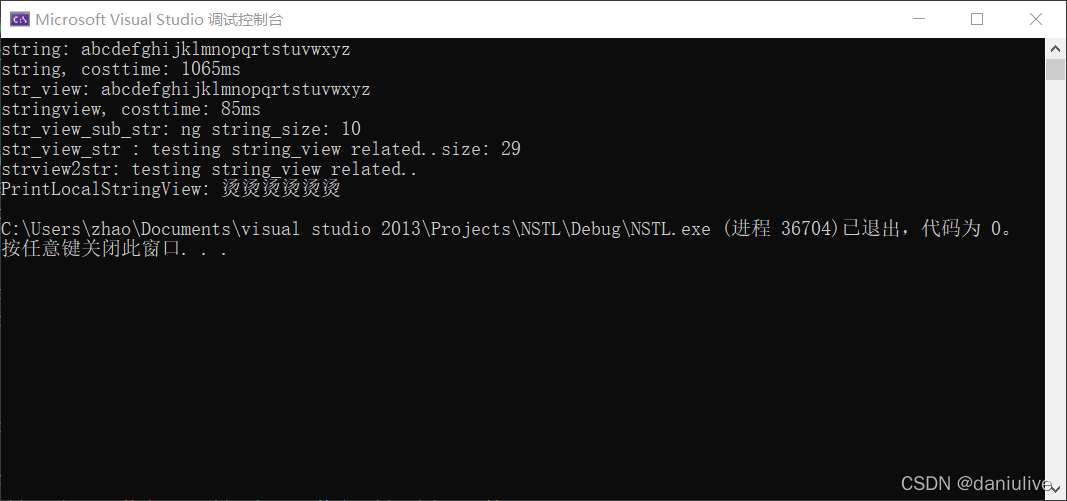# 透彻理解C++11新特性：右值引用、std::move、std::forward

+关注继续查看

C++11出现的右值相关语法可谓是很多C++程序员难以理解的新特性，不少人知其然而不知其所以然，面试被问到时大概就只知道可以减少开销，但是为什么减少开销、减少了多少开销、什么时候用...这些问题也不一定知道，于是我写下了这篇夹带自己理解的博文，希望它对你有所帮助。

class Vector{

int num;
int* a;

public:

void ShallowCopy(Vector& v);
void DeepCopy(Vector& v);

};

//浅拷贝
void Vector::ShallowCopy(Vector& v){

this.num = v.num;
this.a = v.a;

}

//深拷贝
void Vector::DeepCopy(Vector& v){

this.num = v.num;
this.a = new int[num];
for(int i=0;i<num;++i){a[i]=v.a[i]}

}

a = ++b;
a = b+c*2;
a = func();

//左值引用形参=>匹配左值
void Vector::Copy(Vector& v){

this.num = v.num;
this.a = new int[num];
for(int i=0;i<num;++i){a[i]=v.a[i]}

}

//右值引用形参=>匹配右值
void Vector::Copy(Vector&& temp){

this.num = temp.num;
this.a = temp.a;

}

//拷贝构造函数：这意味着深拷贝
Vector::Vector(Vector& v){

this.num = v.num;
this.a = new int[num];
for(int i=0;i<num;++i){a[i]=v.a[i]}

}
//移动构造函数：这意味着浅拷贝
Vector::Vector(Vector&& temp){

this.num = temp.num;
this.a = temp.a;
temp.a = nullptr;    //实际上Vector一般都会在析构函数来释放指向的内存，所以需赋值空地址避免释放

}

void func(){

Vector result;
//...DoSomehing with ans
if(xxx){ans = result;}  //现在我希望把结果提取到外部的变量a上。
return;

}

void func(){

Vector result;
//...DoSomehing with ans
if(xxx){ans = std::move(result);}   //调用的是移动赋值构造函数
return;

}

void test(Vector& o) {std::cout << "为左值。" << std::endl;}
void test(Vector&& temp) {std::cout << "为右值。" << std::endl;}

int main(){

Vector a;
Vector&& b = Vector();
//请分别回答：a、std::move(a)、b 分别是左值还是右值？
test(a);
test(std::move(a));
test(b);

}

//即使函数返还值是临时值，但返还类型是左值引用类型，因此被认为是持久存在的左值。
Vector& func1();
//函数返还值为右值引用类型=>是短暂存在的右值。
Vector&& func2();
//函数返还值为正常类型=>是短暂存在的右值。
Vector func3();

void func1(Vector v) {return;}
void func2(Vector && v) {return;}

int main() {

Vector a;
Vector &b = a;
Vector c;
Vector d;

//请回答：不开优化的版本下，调用以下函数分别有多少Copy Consturct、Move Construct的开销？
func1(a);
func1(b);
func1(std::move(c));
func2(std::move(d));

}

Vector func1() {

Vector a;
return a;

}

Vector func2() {

Vector a;
return std::move(a);

}

Vector&& func3() {

Vector a;
return std::move(a);

}

int main() {

//请回答：不开优化的版本下，执行以下3行代码分别有多少Copy Consturct、Move Construct的开销？
Vector test1 = func1();
Vector test2 = func2();
Vector test3 = func3();

}

template
void func(T&& t){

return;

}

int main() {

Vector a,b;
func(a);                //OK
func(std::move(b));     //OK

}

template
void f(T&& value);

void f(int a);
//当调用f(long类型的参数)或者f(short类型的参数)，则不会匹配int版本而是匹配到万能引用的版本

template
void func(T&& t){

return;

}

int main(){

func(Vector()); //模板参数T被推导为Vector&&

}

& & &
&& & &&
Example1：

func(Vector());

Example2：

func(a);

//当然我们也可以写成如下重载代码，但是这已经违背了使用万能引用的初衷（仅编写一个模板函数就可以匹配左值、右值）
template
void func(T& t){

doSomething(t);

}

template
void func(T&& t){

doSomething(std::move(t));

}

template
void func(T&& object){

doSomething(std::forward<T>(object));

}

std::forward()的实现主要就一句return static_cast(形参)，实际上也是利用了折叠规则。从而接受右值引用类型时，将右值引用类型的值返还（返还值为右值）。接受左值引用类型时，将左值引用类型的值返还（返还值为左值）。|
21天前
|

C++14新特性扫盲探究

26 0
|
21天前
|

C++17新特性之try_emplace与insert_or_assign

11 0
|
21天前
C++17新特性之std::string_view
std::string_view系C++17标准发布后新增的内容，类成员变量包含两个部分：字符串指针和字符串长度，相比std::string, std::string_view涵盖了std::string的所有只读接口。如果生成的std::string无需进行修改操作，可以把std::string转换为std::string_view，std::string_view记录了对应的字符串指针和偏移位置，无需管理内存，相对std::string拥有一份字符串拷贝，如字符串查找和拷贝，效率更高。
11 0|
21天前
|

C++11新特性探究：显式override和final
C++中，我们一般可以以基类声明纯虚函数，然后让派生类继承并重写这个虚函数，用override表示显示覆盖基类方法，但一直没有提供一种方法来阻止派生类继承基类的虚函数。
14 0
|
21天前
|
C++
C++11新特性探索：原始字符串字面值(raw string literal)

16 0
|
1月前
|

2023-4-6-C++11、C++14、C++17、C++20版本新特性系统全面的学习！（四）
2023-4-6-C++11、C++14、C++17、C++20版本新特性系统全面的学习！
59 0
|
1月前
|

2023-4-6-C++11、C++14、C++17、C++20版本新特性系统全面的学习！（三）
2023-4-6-C++11、C++14、C++17、C++20版本新特性系统全面的学习！
40 0
|
1月前
|

2023-4-6-C++11、C++14、C++17、C++20版本新特性系统全面的学习！（一）
2023-4-6-C++11、C++14、C++17、C++20版本新特性系统全面的学习！
82 0
|
3月前
|

C++11之右值引用：移动语义和完美转发(带你了解移动构造函数、纯右值、将亡值、右值引用、std::move、forward等新概念)
C++11之右值引用：移动语义和完美转发(带你了解移动构造函数、纯右值、将亡值、右值引用、std::move、forward等新概念)
50 0